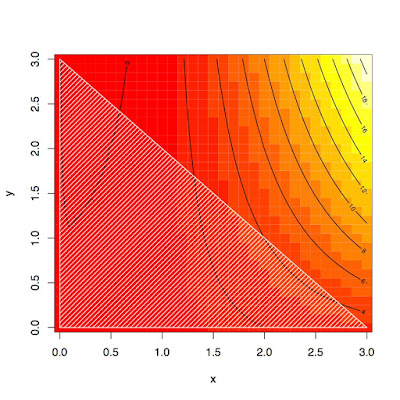## Monday, September 07, 2015

### Nonlinear constraints with a modified constrOptim

I often use constrOptim to quickly solve nonlinear optimization problems. constrOptim works well as a general tool to tackle constrained problems like
$\min_{Ax -b \geq0} f(x)$
There are many other options and packages for specific problems but constrOptim is likely to be the first choice when little is known on the problem at hand or for exploratory/quick-and-dirty analysis.

The main problem I have with it is the nature of the constraints: they must be linear (written in matrix form as $$Ax-b≥ 0$$). Often I would like to compute (local) solutions of more general problems of the form
$\min_{g(x) -b \geq0} f(x)$
where g(x) takes vector values, one component for each scalar constraint, and is in general nonlinear, i.e., $$g(x)$$ cannot be written as $$Ax$$.

Hence, I tweaked the code of constrOptim replacing all the occurrences of the linear constraints with my "new" non linear constraints. (In order to do that, I had to discard the chance to use "BFGS" as a solving method, for now...)  The result is a function, called constrOptimNL, see the code below, which can handle non linear constraints if a vector function g(x) and b are provided. All the drawbacks of the old function are inherited, that's life, but now non linear problems can be solved as shown by the following simple but non entirely trivial example in two dimensions.
 f <- function(x,y) x^2*y+x-x*y
fb <- function(x) f(x,x) #vectorial version of f
x <- seq(0,3,length=31)
y <- seq(0,3,length=31)
z <- outer(x,y,f)
#draws a graph
polygon(c(0,0,3),c(0,3,0),col="white",density=20)Heatmap and contour lines of the objective function, the feasible domain is filled in white.

Consider the constraints $$x\geq0,y\geq 1, x+y\leq 3$$, the feasible domain is a triangle with vertices (0,0), (0,3), (3,0): due to linearity, the problem can be solved with

 A <- matrix(c(1,0,-1,0,1,-1),3,2)
b <- c(0,1,-3)
res <- constrOptim(c(0.5,1.5),fb,NULL,A,b)
res
$par  0.2792393 2.7207607$value
 -0.2683538
$counts function gradient 246 NA  ... The same problems can be solved with constrOptimNL, which trivially can handle linear constraints as well:  g <- function(x,y) c(x,y,-x-y) gb <- function(x) g(x,x) source("constrOptimNL.R") resNL <- constrOptimNL(c(0.5,1.5),fb,NULL,gb,b) resNL $par
 0.2792393 2.7207607
$value  -0.2683538$counts
246 NA
... 

Assume now that some non linear constraint is involved in the optimization problem: $$x\geq0,y\geq1,(x-1)^2+(y-1)^2\leq1$$, the feasible domain is now the upper half-circle centered at (1,1) with unit radius. This problem has nonlinearities in the constraints and cannot be solved with the standard constrOptim.
 g <- function(x,y) c(x,y,-(x-1)^2-(y-1)^2)
b <- c(0,1,-1)
resNL <- constrOptimNL(c(0.5,1.5),fb,NULL,gb,b)
resNL$par   0.2649931 1.6780596  A graph shows that this is indeed the correct solution:  alpha <- seq(0,pi,len=31) image(x,y,z); contour(x,y,z,add=T) polygon(cos(alpha)+1,sin(alpha)+1,col="white",density=20) #draws the half-circle points(resNL$par,resNL$par)Contour levels and a nonlinear feasible domain in white (the solution is plotted with a filled circle). You can check the solution more formally substituting the nonlinear constraint in polar coordinates, $$x=\cos\alpha+1,y=\sin\alpha+1$$:  f2 <- function(alpha) f(cos(alpha)+1,sin(alpha)+1) curve(f2,0,pi) sol <- optimize(f2,c(0,3)) points(sol$minimum,sol$objective) c(cos(sol$minimum)+1,sin(sol$minimum)+1) #"same" as resNLObjective function restricted to the half-circle ### The code The code for constrOptimNL is given below (in this version the parameter grad is kept for compatibility with constrOptim but will never be used: Nelder-Mead and SANN are derivatives free and invoking BFGS stops the function). Hope this helps.  constrOptimNL <- function (theta, f, grad, g, ci, mu = 1e-04, control = list(), method = if (is.null(grad)) "Nelder-Mead" else "BFGS", outer.iterations = 100, outer.eps = 1e-05, ..., hessian = FALSE) { if(method=="BFGS") stop("method BFGS not available, use Nelder-Mead or SANN") if(!is.null(control$fnscale) && control$fnscale < 0) mu <- -mu R <- function(theta, theta.old, ...) { ui.theta <- g(theta,...) gi <- ui.theta - ci if (any(gi < 0)) return(NaN) gi.old <- g(theta.old,...) - ci bar <- sum(gi.old * log(gi) - ui.theta) if (!is.finite(bar)) bar <- -Inf f(theta, ...) - mu * bar } dR <- function(theta, theta.old, ...) { ui.theta <- g(theta,...) gi <- drop(ui.theta - ci) gi.old <- drop(g(theta.old,...) - ci) dbar <- colSums(ui * gi.old/gi - ui) grad(theta, ...) - mu * dbar } if (any(g(theta,...) - ci <= 0)) stop("initial value is not in the interior of the feasible region") obj <- f(theta, ...) r <- R(theta, theta, ...) fun <- function(theta, ...) R(theta, theta.old, ...) gradient <- if (method == "SANN") { if (missing(grad)) NULL else grad } else function(theta,...) dR(theta, theta.old, ...) totCounts <- 0 s.mu <- sign(mu) for (i in seq_len(outer.iterations)) { obj.old <- obj r.old <- r theta.old <- theta a <- optim(theta.old, fun, gradient, control = control, method = method, hessian = hessian, ...) r <- a$value
if (is.finite(r) && is.finite(r.old) && abs(r - r.old) <
(0.001 + abs(r)) * outer.eps)
break
theta <- a$par totCounts <- totCounts + a$counts
obj <- f(theta, ...)
if (s.mu * obj > s.mu * obj.old)
break
}
if (i == outer.iterations) {
a$convergence <- 7 a$message <- gettext("Barrier algorithm ran out of iterations and did not converge")
}
if (mu > 0 && obj > obj.old) {
a$convergence <- 11 a$message <- gettextf("Objective function increased at outer iteration %d",
i)
}
if (mu < 0 && obj < obj.old) {
a$convergence <- 11 a$message <- gettextf("Objective function decreased at outer iteration %d",
i)
}
a$outer.iterations <- i a$counts <- totCounts
a$barrier.value <- a$value
a$value <- f(a$par, ...)
a$barrier.value <- a$barrier.value - a\$value
a
}